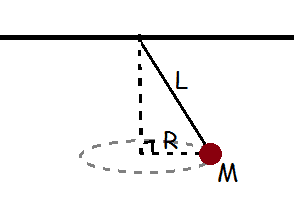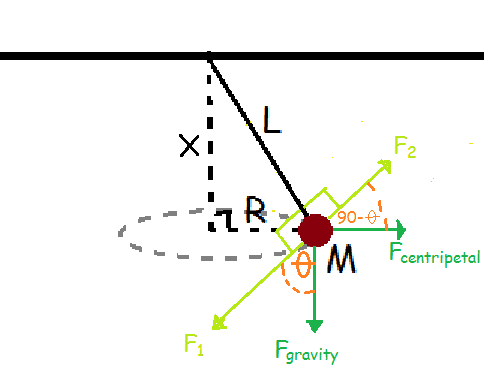# Kinematics and dynamic circular motion of conical pendulum

## Homework Statement[/B]
find the period with only using L (for the long of the rope), R (for the radius), M (for the mass), and G (for the gravity)

## Homework Equations

V=ωR
Fcentripetal = ##\frac {MV^2} {R}##
Fgravity = MG
phytagoras
basic trigonometry

## The Attempt at a Solution[/B]
i have tried to do it this way
##x=\sqrt {L^2 - R^2}##
##F_1=F_2##
##MG Cos (θ) = \frac {MV^2} {R} Cos (90-θ) ##
##\frac {MGR} {L} = \frac{MV^2 \sqrt {L^2 - R^2}} {RL}##
##\frac {MGR} {L} = \frac{Mω^2 R^2 \sqrt {L^2 - R^2}} {RL}##
##\frac {MGR} {L} = \frac{M4π^2 R^2 \sqrt {L^2 - R^2}} {RLT^2}##
##T^2 = \frac{4π^2 \sqrt {L^2 - R^2}} {G}##
##T = \sqrt {\frac{4π^2 \sqrt {L^2 - R^2}} {G}}##
am i right?
some of my friend have i different answer from me, actually, i dont really know where is the centripetal force direction

can someone explain me what is centripetal force actually with answering this question

Hi,
I think your solution is correct.
Centripetal acceleration is acceleration which makes something 'go in a circle' - not straight forward. It points toward the centre of the circle.
Multiplied with the mass, this gives the 'centripetal force'.
Hope this helped.

Hi,
I think your solution is correct.
Centripetal acceleration is acceleration which makes something 'go in a circle' - not straight forward. It points toward the centre of the circle.
Multiplied with the mass, this gives the 'centripetal force'.
Hope this helped.
ok, thank you very much